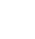# Loop through worksheets and extract specific cells to a new worksheet

There may be an instance where you have potentially hundreds or even thousands of worksheets in a workbook, or have collated a large number of identical worksheets together and need to extract specific cells in each sheet and store them in a table or each sheets own row on 1 main worksheet.

The VBA script below is a basic example of how this can be achieved. It starts by declaring variables, and then we set OTP as 2 which will be the first row we want to put output data into. You will need to have a worksheet named ‘Output’ as the second tab, and all your source tabs from tab 3 onwards – thousands if applicable.

The code opens each worksheet from tab 3 onwards and takes the value in A2, putting it in the Output tab in A2. Then it takes the value in A2 of the source and puts it in B2 and so on. You will need to amend the code (far right where it says A2) to pinpoint the cell you want to grab. Before we start the loop again and move on to the next tab and grab the same data, we need to increment OTP by 1 so that the next row in the Output tab takes the info from the next tab.

We’d be happy to help create a bespoke system for you where you can use the mouse to click and select any number of cell locations on a sheet and apply it to this example, grabbing all the data you need into a row. Contact us below or leave a message.

``` Sub Extract_All_to_own_row() Dim WS_Count As Integer Dim I As Integer```

``` OTP = 2 ' Set WS_Count equal to the number of worksheets in the active workbook. WS_Count = ActiveWorkbook.Worksheets.Count ' Begin the loop. For I = 3 To WS_Count Worksheets("Output").Range("A" & OTP) = ActiveWorkbook.Worksheets(I).Range("A2") Worksheets("Output").Range("B" & OTP) = ActiveWorkbook.Worksheets(I).Range("A2") Worksheets("Output").Range("C" & OTP) = ActiveWorkbook.Worksheets(I).Range("A2") Worksheets("Output").Range("D" & OTP) = ActiveWorkbook.Worksheets(I).Range("A2") Worksheets("Output").Range("E" & OTP) = ActiveWorkbook.Worksheets(I).Range("A2") Worksheets("Output").Range("F" & OTP) = ActiveWorkbook.Worksheets(I).Range("A2") Worksheets("Output").Range("G" & OTP) = ActiveWorkbook.Worksheets(I).Range("A2") Worksheets("Output").Range("H" & OTP) = ActiveWorkbook.Worksheets(I).Range("A2") Worksheets("Output").Range("I" & OTP) = ActiveWorkbook.Worksheets(I).Range("A2") Worksheets("Output").Range("J" & OTP) = ActiveWorkbook.Worksheets(I).Range("A2") Worksheets("Output").Range("K" & OTP) = ActiveWorkbook.Worksheets(I).Range("A2") Worksheets("Output").Range("L" & OTP) = ActiveWorkbook.Worksheets(I).Range("A2") Worksheets("Output").Range("M" & OTP) = ActiveWorkbook.Worksheets(I).Range("A2") OTP = OTP + 1 Next I End Sub ```